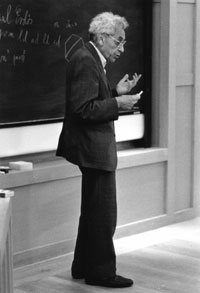# Large Girth and Chromatic NumberUncle Paul

Paul Erdös is an inspiring figure.  Not only for mathematicians and computer scientists but for everyone. His simplicity, his love for his friends, for music and of course for mathematics indicate a man who followed his feelings which had the word love in them. If you get to meet people that knew him personally and well you will see how much they love him and keep his memory alive. Anyway, this post is about one of his gems. The proof of the existence of graphs with large girth and chromatic number. For completeness, let us mention that the girth of a graph$G$ is the length of the shortest cycle in$G$. The chromatic number of$G$ is the minimum number of colors needed to color properly the vertices of$G$, i.e., no two adjacent vertices get the same color.  First,  try to think the problem on your own and notice that the existence of such graphs is not obvious at all.  The gem of Erdös follows.

Theorem: For any positive integers$k,l$ there exists a graph with girth$\geq l$ and chromatic number$\geq k$.

The proof introduces techniques, therefore let us take a close look at it.

Proof: Pick a random graph$G$ from$G(n,p)$ for$p= n^{1/l-1}$.   Note that we are above the connectivity  threshold which is$\frac{\log{n}}{n}$. Let$X$ be the number of cycles of length$.  Let’s compute the expectation of$X$. The probability of a cycle of length$i$ is clearly$p^i$ for any$i=3,..,l-1$. To finish the computation we need to count how many cycles of length$i$ exist. First we need to pick the$i$ vertices. There are${n \choose i}$ ways to do so. Now we have our$i$ vertices and we need to put them in a cycle.  Clearly there are$i!$ ways to do so but notice that we have to divide by$2 \times i$ since we don’t care about the starting position (we have a cyclic permutation) nor about the orientation. Now we can write down the expectation:$E(X) = \sum_{i=3}^{l-1} {n \choose i} \frac{i!}{2i} p^i$.  As a simple exercise try to show that$E(X) = o(n)$. Given the latter fact,$Pr(X \geq n/2) =o(1)$ by Markov’s inequality.

Now we turn our attention to the independent set of maximum size in$G$. Let that size be$I$ and the chromatic number$C$.  The reason is that there is a straight-forward connection between$C,I$, namely$C \geq \frac{n}{I}$.  Since our graph is sparse we expect to be able to find a large independent set but how large can it be? With some foresight by setting$z=\frac{3\log{n}}{p}$, it is straight-forward to show that$Pr(I \geq z)=o(1)$.

Hence, we have shown so far two facts:$Pr(X \geq n/2) =o(1)$,$Pr(I \geq \frac{3\log{n}}{p})=o(1)$. Hence there exists a graph$G$ such that it has less than n/2 cycles of length <l and also it’s maximum independent set has size < 3log(n)/p.

Now here is a neat trick: we kill the cycles by removing one vertex from each cycle. Therefore we force the girth of the resulting graph to be$\geq l$. Furthermore, the number of vertices we removed was less than$n/2$ and therefore we are left with at least$n/2$ vertices.  Also the size of the maximum independent set cannot have increased! Therefore, you can now check that the chromatic number is at least$\frac{n^{1/l}}{6log{n}} \rightarrow +\infty$ as$n\rightarrow +\infty$.

Q.E.D.

You can find the original article in this link